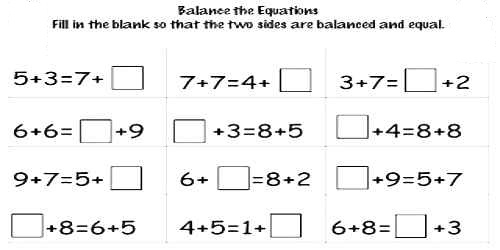To solve an equation means to find a value for the variable that makes the equation true. Whatever you do to one side of the equation, you must also do to the other side. Solving an equation is like solving a puzzle! It means finding a value for the variable that makes the equation true.

An equation is a mathematical statement that has an expression on the left side of the equals sign (=) with the same value as the expression on the right side. An example of an equation is 2 + 2 = 4.

One of the terms in an equation may not be known and needs to be determined. The unknown term may be represented by a letter such as x (e.g. 2 + x = 4). The equation is solved by finding the value of the unknown x that makes the two sides of the equation have the same value.

Use the subtractive equation property to find the value of x in addition equations. The subtractive equation property states that the two sides of an equation remain equal if the same number is subtracted from each side.

Explanation

First, let’s look at a simple addition equation, x + 15 = 30.

To solve the equation we must try to get the variable x alone on one side. We can use the inverse of adding 15 – or subtracting 15 – to get x alone on the left side. Now we have x alone on the left side, since 15 – 15 = 0, but the scale is not in balance. To balance the scale, we must also subtract 15 from the right side of the equation.

x + 15 – 15 = 30 – 15, so, x = 15

30 – 15 = 15, so we find that x = 15.Example:

5 + x = 12

5 + x – 5 = 12 – 5

0 + x = 7

x = 7

Check the answer by substituting (7) for x in the original equation. The answer is correct if the expressions on each side of the equals sign have the same value.

5 + 7 = 12

Information Source: